# Vaex – Faster Pandas Alternate in PythonVaex Python is an alternative to the Pandas library that take less time to do computations on huge data using Out of Core Dataframe. It has fast, interactive visualization capabilities as well.

Pandas is the most widely used python library for dealing with dataframes and processing. The popularity is due to the convenient, easy to understand API it offers along with wide variety of tools. But then, pandas has it shortcomings and a alternative is Vaex. Let’s find out exactly why!## 1. Why do we need Vaex ?

Pandas is a python library used extensively for reading csv files and processsing dataframes. While pandas works smoothly while dealing with smaller data, it becomes very slow and inefficient when there are huge datasets.

Nowadays, it has become very common to encounter datasets that are larger than the available RAM on your system. In cases like these, pandas can’t help you. Also, the complex groupby operations are very slow in pandas. It also does not support memory mapped datasets.

What is the solution we need for this ?

We need a solution that can resolve all the above problems while still providing a convenient API. That solution is nothing but Vaex !

In the upcoming sections, I shall tell you what exactly Vaex is and why is it an alternative to pandas.

## 2. What is Vaex ?

Vaex is a python library that is closely similar to Pandas. Vaex is a library especially for lazy Out-of-Core DataFrames, helps to visualize and explore big tabular datasets. It is a high performance library and can solve many of the shortcomings of pandas. As the API is similar to pandas, users do not face difficulty in shifting. It’s also integrated with Jupyter which makes it easy.

Vaex is capable to calculate statistics such as mean, standard deviation etc, on an N-dimensional grid up to a billion (109109) objects/rows per second. It can also help in the Visualization using histograms, density plots and 3d volume rendering, allowing interactive exploration of big data.

Vaex achieves this high performance through the combination of memory mapping, a zero memory copy policy, and lazy computations, etc. Don’t worry if you these terms go over your head. I shall explain each of them in detail with examples.

First, install and import the python library as shown below.

# !pip install vaex
import vaex


## 3. Vaex uses Memory mapping for large datasets

As we discussed previously, vaex is very useful in case of huge tabular datasets. Let’s say we have a dataset that is larger than the RAM available. How can you load this using vaex ?

Vaex uses Memory mapping to solve this. All the dataset files read into vaex are memory mapped.

## Want to become awesome in ML?

Hi! I am Selva, and I am excited you are reading this!
You can now go from a complete beginner to a Data Science expert, with my end-to-end free Data Science training.
No shifting between multiple books and courses. Hop on to the most effective way to becoming the expert. (Includes downloadable notebooks, portfolio projects and exercises)

Start free with the first course 'Foundations of Machine Learning' - a well rounded orientation of what the field of ML is all about.

Enroll to the Foundations of ML Course (FREE)

When you open a memory mapped file with Vaex, you don’t actually read the data. Vaex will swiftly reads the file metadata (like the location of the data on disk, number of rows, number of columns, column names and types), the file description. So, you can open these files quickly, irrespective of how much RAM you have. But remember that format of memory mappable files are Apache Arrow , HDF5, etc.

Let’s see an example. You can download the dataset I’m using from here

# Reading data from local disk
df=vaex.open('yellow_tripdata_2020-01.hdf5')


But many times, the data available is in the form of CSV files. In these cases, you will have to convert the CSV data into HDF5 format.

How to convert a csv file to hdf5 using vaex?

We have a big csv file here. You can use the vaex.from_csv() function to load in csv files. There is a parameter convert to decide if you want to convert it into HDF5 or not. In this case, we go for convert=True.

Vaex will read the CSV in chunks, and convert each chunk to a temporary HDF5 file which is further concatenated into a single HDF5 file.You can decide the size of the individual chunks using chunk_size argument.

# Converting csv into HDF5 and reading dataframe
%time df = vaex.from_csv('yellow_tripdata_2020-01.csv', convert=True)
df


Wall time: 1.43 sYou can see that it took merely 1.43 seconds ! Lets see how much time it would have taken using normal pandas.

import pandas as pd


Wall time: 2min 34s


It took 2min 34 seconds, which is so slow compared to using vaex. I hope you understood how much time memory mapping can save through this comparison.

## 4. Vaex is lazy : Saves memory

We know that Vaex is very similar to the pandas API. But, there is a fundamental distinction between vaex and pandas.

Vaex is lazy.

That means, vaex does not actually perform the operation or read through whole data unless necessary (unlike pandas). For example, say you call an expression like: df['passenger_count'].mean, the actual computations does not happen. It just notes down what computations it must do. A vaex expression object is created instead, and when printed out it shows some preview values. This significantly saves memory space.

df['passenger_count'].mean


Let’s have a look at another lazy computation example.

import numpy as np
np.sqrt(df.passenger_count**2 + df.trip_distance**2)

Expression = sqrt(((passenger_count ** 2) + (trip_distance ** 2)))
Length: 6,405,008 dtype: float64 (expression)
---------------------------------------------
0  1.56205
1  1.56205
2  1.16619
3  1.28062
4        1
...
6405003      nan
6405004      nan
6405005      nan
6405006      nan
6405007      nan


With the expression system, vaex performs calculations only when needed. Also, the data does not need to be local, expressions can be sent over a wire, and statistics can be computed remotely, something that the vaex-server package provides.

Let’s move ahead to other interesting features with vaex. You’ll be able to observe the “lazy computation” is a main foundation behind many of them.

## 5. Virtual Columns

When you write expressions to create a new column in vaex dataframe, a virtual colum is created.

But what is a virtual column?

A virtual column behaves just like a regular column but occupies no memory. Why is this so ?

This is because Vaex only remembers the expression the defines them. It does not calculate the values up front like pandas.This saves both memory and time .These columns are lazily evaluated only when it is necessary, keeping memory usage low.

Let’s look at an example.

Consider the dataframe df we loaded in previous section. we’ll use the same here. Let’s write an expression to create a new column new_trip_distanceas per the below expression. This column will now be a virtual column and no memory alotted. Let’s record the time taken too.

%time df['new_trip_distance'] = df['trip_distance'] + 10

Wall time: 998 µs


The task was completed in microseconds because there was no need to allot memory. Lets see how much time we saved by performing the same task on the pandas dataframe. Check below code and time.

%time pandas_df['new_trip_distance'] = pandas_df['trip_distance'] + 10

Wall time: 1.34 s


It took almost 1500x more time for this!

Also, this virtual column new_trip_distnace is lazily evaluated on the fly when required.

dfI hope you understood how virtual columns work, let’s move ahead to other operations and features

## 6. Data cleansing with Vaex

Data cleaning and filtering are the crucial steps that often take up a lot of time in python. For example, let’s take the same dataframe we used in previous sections. Say you wish to filter out the records whose passenger_count is greater than 10. Let’s try it using the normal pandas and see how much time it takes.

%time df_filtered=pandas_df[pandas_df['passenger_count']>10]

Wall time: 13.6 s


You can see that it’s slow. Let’s see perform the same task on the vaex dataframe.

%time df_filtered=df[df['passenger_count']>10]

Wall time: 611 ms
Parser   : 106 ms


Vaex reduced the time taken from 13.6 seconds to micro seconds!

Hoe did vaex manage to do that ?

It is because of the zero memory copy policy followed by vaex. This means that filtering a DataFrame costs very little memory and does not copy the data. df_filtered has a ‘view’ on the original data. Even when you filter a 1TB file, just a fraction of the file will be read. This means that when you have large no of missing values, you can drop them or fill them at almost no cost.

%time df_fillna=df.fillna(value=0, column_names=['passenger_count'])
df_fillna


Wall time: 483 msVaex did the painful task very quickly for us.

## 7. Statistics performance : Vaex vs Pandas

Vaex is very popular for the high performance it offers when it comes to statistics. While dealing with big tabular datsets ,you will need a alternative to pandas’s groupby. You need a solution that is computationally much faster.So, Vaex alllows you to perform statistics on a regular N-dimensional grid, which is blazing fast. It has been proven that Vaex can calculate the mean of about a billion row data in just a second !

Below is an example of efficient calculation of statistics on N-dimensional grids

# Every statistic method accepts a binby argument to compute statistics on regular Nd array
df.mean(df.passenger_count, binby=df.DOLocationID, shape=20)

array([1.53489408, 1.49914832, 1.49319968, 1.54545849, 1.49560378,
1.52010031, 1.50486626, 1.52510748, 1.51555149, 1.55267282,
1.50574786, 1.5412169 , 1.50043236, 1.48509443, 1.52030571,
1.53979913, 1.48159731, 1.51295217, 1.51658428, 1.52362767])


Now let’s compare some statistic computations of pandas and vaex.

Below, let’s try and calculate the mean of any column using both pandas and vaex.

%time df.mean(df.fare_amount)

Wall time: 769 ms

array(12.69410812)

%time pandas_df['fare_amount'].mean()

Wall time: 1.64 s

12.69410811978051


Vaex was 3X times faster in above case

## 8. Selections

In the previous section, we saw how strong vaex was in statistics. Let’s explore another interesting feature offered by vaex : Selections .

A Selection is used to define a subset of the data. This helps in two ways. Firstly, it helps to filter te data from the dataframe quick. Apart from this , selections enable you to calculate the statistics for multiple subsets in a single pass over the data. We can do multiple steps in a single line, that too amazingly fast! This application is very useful especially while dealing with DataFrames that don’t fit into memory (Out-of-core).

Let’s understand how to use selections with an example. Say for the previous dataframe of New york taxi data, we need to create subsets based on no of passengers and find the mean fare amount for each subset.Using selection, it can be done in a si gle line as shown below.

df.mean(df.fare_amount,selection=[df.passenger_count<2,df.passenger_count>2])

array([12.38094964, 12.6061761 ])


You might have also noticed that it was very quick! Because, vaex does not copy the data like pandas. What does it do then ?Vaex internally keeps track which rows are selected.

Apart from this, there is another main use-case of the bin computation and the selections feature : they make visualization faster and easier! Let’s learn about them in the next section.

## 9. Fast Visualizations with Vaex

Visualizations are a crucial part to understanding the data we have. It gives a clear result to picture the trends and derive insights. But when you have huge data frame of million rows, making standard scatter plots takes a really long time. Not only that, but the visualizations are illegible and not clear. What is the solution here ?

Again, Vaex saves the day!

With the help of group aggregations, selections and bins, vaex can compute these visualizations pretty quickly. Most of the visualizations are done in 1 or 2 dimensions. Also and Vaex nicely wraps Matplotlib so that python users are convenient. We shall see some examples of fast visualizations in this section.

### 1D PLOTS

Consider the dataframe used previously. Let’s say we need to visualize the values taken by fare_amount. You can easily visualize through a 1D plot by making use of vaex’s plot1d() function. There is a parameter limits that will show a histogram showing 99.7% of the data as shown below.

%time df.plot1d(df.fare_amount,limits='99.7%')

Wall time: 404 ms

[]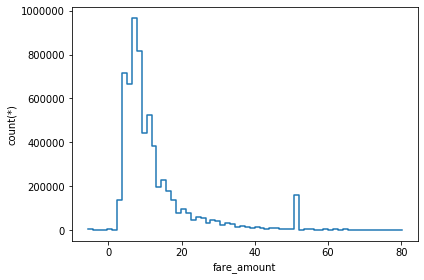### 2D PLOTS

We can also visualize the data in a 2D histogram or heatmap. The DataFrame.plot() function is used for this.
Now, let’s try and to plot a 2D plot using the same dataframe on NYC taxi data. Check below code.

df.plot(df.total_amount , df.trip_distance, limits=[-20,20])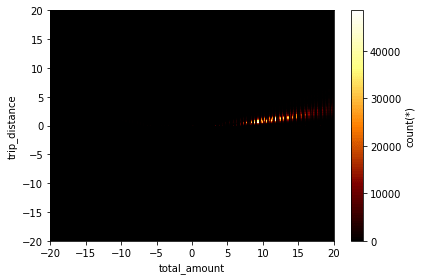Let us look at a few more examples. For this, I will be using the example dataframe inbuilt in vaex. You can simply load it bu calling vaex.example(). Below is the view to this dataframe.

df_example = vaex.example()
df_exampleLet’s create a 2D plot using this df_example. An amazing feature vaex offers is the what parameter of the plot() function. You can define the mathematical relation which has to be plotted(shape equals length of what argument). Below is an example of 2D plotting

df_example.plot(df_example.x, df_example.y, what=vaex.stat.mean(df_example.E)**2, limits='99.7%')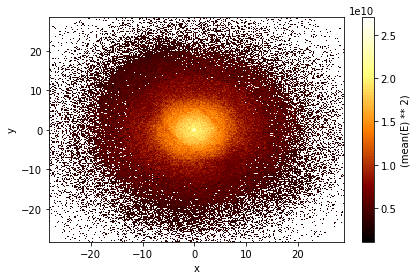Selections for plotting

Previously, we saw that vaex uses selections to speed up filtering. These also help in fast visualizations. Instead of filtering and having 4 different columns like in pandas, you can have 4 (named) selections in your DataFrame. Now, you can calculate statistics in just one single pass over the data. This is significantly faster especially in the cases when your dataset is larger than your RAM. Let’s see an example below. I have plotted using three selections.

df_example.plot(df_example.x, df_example.y, what=np.log(vaex.stat.count()+1), limits='99.7%',
selection=[None, df_example.x < df_example.y, df_example.x < -10]);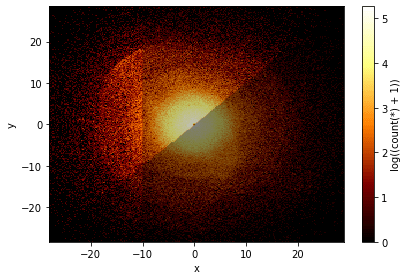You can see that, by default the graphs are faded on top of each other type. If you want it as separate column , then you can pass the option through the visual parameter. This Will plot each selection as a column. See below example

import numpy as np
df_example.plot(df_example.x, df_example.y, what=np.log(vaex.stat.count()+1), limits='99.7%',
selection=[None, df_example.x < df_example.y, df_example.x < -10],visual=dict(column='selection'))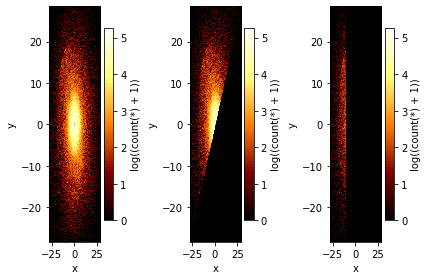## Similar Articles

### Logistic Regression – A Complete Tutorial With Examples in RCourse Preview

## Machine Learning A-Z™: Hands-On Python & R In Data Science

### Free Sample Videos:#### Machine Learning A-Z™: Hands-On Python & R In Data Science#### Machine Learning A-Z™: Hands-On Python & R In Data Science#### Machine Learning A-Z™: Hands-On Python & R In Data Science#### Machine Learning A-Z™: Hands-On Python & R In Data Science